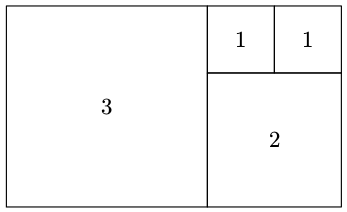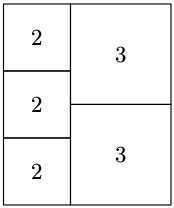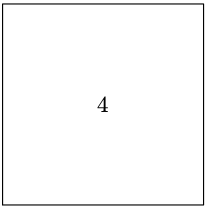### H - Hard Cuts

##### Languages: C, C++, Java, JavaScript, ... (details)

Given a rectangle with integer side lengths, your task is to cut it into the smallest possible number of squares with integer side lengths.

#### Input

The first line contains a single integer $T$ — the number of test cases ($1 \le T \le 3600$). Each of the next $T$ lines contains two integers $w_i$, $h_i$ — the dimensions of the rectangle ($1 \le w_i, h_i \le 60$; for any $i \ne j$, either $w_i \ne w_j$ or $h_i \ne h_j$).

#### Output

For the $i$-th test case, output $k_i$  the minimal number of squares, such that it is possible to cut the $w_i$ by $h_i$ rectangle into $k_i$ squares. The following $k_i$ lines should contain three integers each: $x_{ij}$, $y_{ij}$  the coordinates of the bottom-left corner of the $j$-th square and $l_{ij}$ — its side length ($0 \le x_{ij} \le w_i - l_{ij}$; $0 \le y_{ij} \le h_i - l_{ij}$). The bottom-left corner of the rectangle has coordinates $(0, 0)$ and the top-right corner has coordinates $(w_i, h_i)$.

#### Sample test(s)

Input
3 5 3 5 6 4 4
Output
4 0 0 3 3 0 2 3 2 1 4 2 1 5 0 0 2 0 2 2 0 4 2 2 0 3 2 3 3 1 0 0 4

#### HintsExample case 1 Example case 2 Example case 3